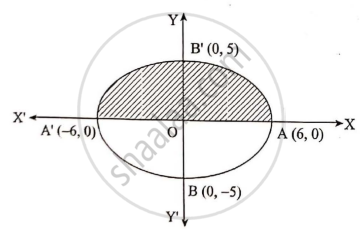# Find the Volume of a Solid Obtained by the Complete Revolution of the Ellipse X 2 36 + Y 2 25 = 1 About X-axis. - Mathematics and Statistics

Sum

Find the volume of a solid obtained by the complete revolution of the ellipse x^2/36 + y^2/25 = 1 about X-axis.

#### Solution

From the equation of the ellipse

x^2/36 + y^2/25 = 1

y^2 = 25/36 (36 - x^2)Lel V be the required volume of the solid obtained by revolving the ellipse about major axis i.e. X-axis.

V = pi∫_-6^6 y^2 dx

= ∫_-6^6 25/36(36 - x^2) dx

= (25pi)/36 .2 ∫_-6^6 (36 - x^2) dx ....(by property)

= (25pi)/18 [36x - x^3/3]_0^6

= (25pi)/18 [36(6) - 6^3/3 - 0]

= (25pi)/18

V = 200π cubic units.

Concept: Applications of Definite Integrals
Is there an error in this question or solution?